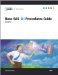# Concepts: RANK Procedure

### Computer Resources

PROC RANK stores all values in memory of the variables for which it computes ranks.

### Statistical Applications

Ranks are useful for investigating the distribution of values for a variable. The ranks divided by n or n +1 form values in the range 0 to 1, and these values estimate the cumulative distribution function. You can apply inverse cumulative distribution functions to these fractional ranks to obtain probability quantile scores, which you can compare to the original values to judge the fit to the distribution. For example, if a set of data has a normal distribution, the normal scores should be a linear function of the original values, and a plot of scores versus original values should be a straight line.

Many nonparametric methods are based on analyzing ranks of a variable:

• A two-sample t -test applied to the ranks is equivalent to a Wilcoxon rank sum test using the t approximation for the significance level. If you apply the t -test to the normal scores rather than to the ranks, the test is equivalent to the van der Waerden test. If you apply the t -test to median scores (GROUPS=2), the test is equivalent to the median test.

• A one-way analysis of variance applied to ranks is equivalent to the Kruskal-Wallis k -sample test; the F-test generated by the parametric procedure applied to the ranks is often better than the X 2 approximation used by Kruskal-Wallis. This test can be extended to other rank scores (Quade 1966).

• You can obtain a Friedman s two-way analysis for block designs by ranking within BY groups and then performing a main-effects analysis of variance on these ranks (Conover 1998).

• You can investigate regression relationships by using rank transformations with a method described by Iman and Conover (1979).Base SAS 9.1 Procedures Guide, Volumes 1, 2, 3 and 4
ISBN: 1590472047
EAN: 2147483647
Year: 2004
Pages: 142